Custom SearchKINETIC THEORY OF GASES

In an attempt to explain the compressibility of gases, Bernoulli proposed the hypothesis that is accepted as the kinetic theory of gases. by continual bombardment of the walls by molecules of the gas.

Consider the container shown in figure 11-2 as containing a gas. At any given time, some molecules are moving in one direction, some are traveling in other directions; some are traveling fast, some slow, and some may even be in a state of rest. The average effect of the molecules bombarding each container wall corresponds to the pressure of the gas.

As more gas is pumped into the container, more molecules are available to bombard the walls; thus the pressure in the container increases. The gas pressure in a container can also be increased by increasing the speed with which the molecules hit the walls. If the temperature of the gas is raised, the molecules move faster causing an increase in pressure. This can be shown by considering the automobile tire. When you take a long drive on a hot day, the pressure in the tires increases and a tire which appeared to be somewhat "soft" in cool morning temperature may appear normal at a higher midday temperature.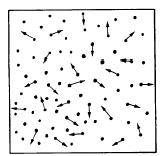Figure 11-2.Molecular bombardment creating pressure.

BOYLES LAW

When the automobile tire is initially inflated, air which normally occupies a specific volume is compressed into a smaller volume inside the tire. This increases the pressure on the inside of the tire. Charles Boyle, an English scientist, was among the first to experiment with the pressure-volume relationship of gas. During an experiment when he compressed a volume of air he found that the volume decreased as the pressure increased, and by doubling the force exerted on the air he could decrease the volume of the air by half. See figure 11-3. Recall from the example of the automobile tire that changes in temperature of a gas also change the pressure and volume. Therefore, the experiment must be performed at a constant temperature. The relationship between pressure and volume is known as Boyles law. It states: When the temperature of a gas is kept constant, the volume of an enclosed gas varies inversely with its pressure.

In equation form, this relationship may be expressed as either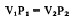or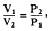Equation 11-6

where V1 and P1 are the original volume and pressure, and V2 and P2 are the final volume and pressure (P1 and P2 are absolute pressures).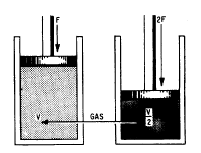Figure 11-3.-Gas compressed to half its original volume by a doubled force.

Example of Boyles law: 4 cubic feet of nitrogen are under a pressure of 100 psi (gauge). The nitrogen is allowed to expand to a volume of 6 cubic feet. What is the new gauge pressure? Remember to convert gauge pressure to absolute pressure by adding 14.7. Using equation 11-6, V1P1 = V2P2, where V1 is 4 ft3, V2 is 6 ft, and P1 is 100 psig: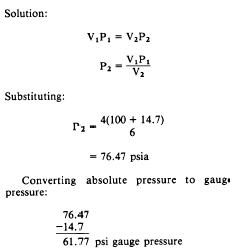CHARLESS LAW

Boyles law assumes conditions of constant temperature. In actual situations this is rarely the case. Temperature changes continually and affects the volume of a given mass of gas. Jacques Charles, a French physicist, provided much of the foundation for the modern kinetic theory of gases. Through experiments, he found that all gases expand and contract proportionally to the change in the absolute temperature, providing the pressure remains constant. The relationship between volume and temperature is known as Charless law. It states: The volume of a gas is proportional to its absolute temperature, if constant pressure is maintained. In equation form, this relationship may be expressed as

Equation 11-7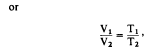where V1 and V2 are the original and final volumes, and T1 and T2 are the original and final absolute temperatures.

Since an increase in the temperature of a gas causes it to expand if the pressure is kept constant, it is reasonable to expect that if a given sample is heated within a closed container and its volume remains constant, the pressure of the gas will increase. Experiments have proven this to be true. In equation form, this becomes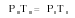or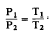Equation 11-8

This equation states that for a constant volume, the absolute pressure of a gas varies directly with the absolute temperature.

Example: A cylinder of gas under a pressure of 1800 psig at 70F is left out in the sun in the tropics and heats up to a temperature of 130F. What is the new pressure within the cylinder? (Remember that both pressure and temperature must be converted to absolute pressure and absolute temperature.)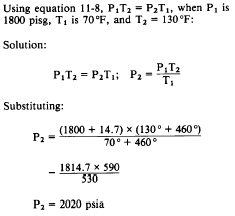Converting absolute pressure to gauge pressure: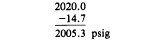GENERAL GAS LAW

We have learned that Boyles law pertains to situations in which the temperature remains constant (fig. 11-4), and that Charless law pertains to situations in which pressure remains constant (fig. 11-4). It is usually not possible to control pressure or temperature in tanks or bottles of gas subject to the weather and shipboard demands. Boyles and Charless laws are combined to form the general gas law. This law states: The product of the initial pressure, initial volume, and new temperature (absolute scale) of an enclosed gas is equal to the product of the new pressure, new volume, and initial temperature. It is a mathematical statement which allows many gas problems to be solved by using the principles of Boyles law and/or Charless law. The equation is expressed as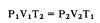or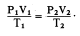(P and T represent absolute pressure and absolute temperature, respectively.) You can see by examining figure 11-4 that the three equations are special cases of the general equation. Thus, if the temperature remains constant, T1 equals T2 and both can be eliminated from the general formula, which then reduces to the form shown in part A. When the volume remains constant, V1 equals V2, thereby reducing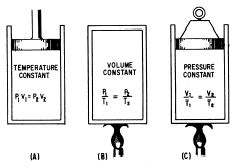Figure 11-4.The general gas law.

the general equation to the form given in part B. Similarly, P1 is equated to P2 for constant pressure, and the equation then takes the form given in part C.

The general gas law applies with exactness only to "ideal" gases in which the molecules are assumed to be perfectly elastic. However, it describes the behavior of actual gases with sufficient accuracy for most practical purposes. Two examples of the general equation follow: 1. Two cubic feet of a gas at 75 psig and 80F are compressed to a volume of 1 cubic foot and then heated to a temperature of 300F. What is the new gauge pressure? Using equation 11-9, P1V1T2 = P2V2T1, where V1 is 2 ft3, P1 is 75 psig, T1 is 80F, V2 is 1 ft3 and T2 is 300F:

Solution: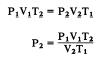Substituting: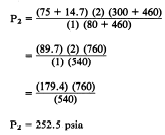Converting absolute pressure to gauge pressure: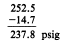2. Four cubic feet of a gas at 75 psig and 80F are compressed to 237.8 psig and heated to a temperature of 300F. What is the volume of the gas resulting from these changes? Using equation 11-9, P1V1T2 = P2V2T1, where V1 is 4 ft3, P2 is T1 is 800, P1 is 237.8 psig, and T2 is 300F:

Solution: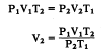Substituting: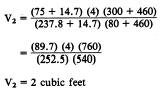Integrated Publishing, Inc. - A (SDVOSB) Service Disabled Veteran Owned Small Business